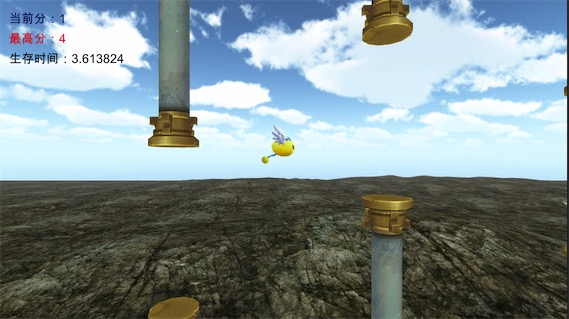### Q_learing

q-learning的伪代码先看这部分，很重要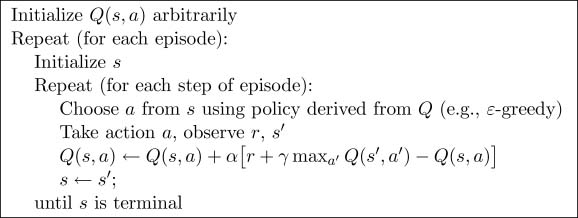• 在当前状态s所有可能的行为中选择一个a
• 利用a得到下一个状态s_
• 计算Q(s,a) (对当前的行为进行学习)
• 下一个状态等于当前状态
• 开始下一个循环

GAMMA(gamma 是对未来 reward（分数） 的衰减值)，ALPHA(学习率)，EPSILON(策略)

GAMMA是什么意思呢，就是对获取过的奖励为了防止再次获取后得到的分数一样，于是对reward进行一个衰减，这样就会有长远的眼光，机器人就不只专注于眼前的奖励了

EPSILON 是一种策略，0.8代表的意思就是我们有80%的概率来选择之前的经验剩下的20%的概率来进行新的探索。

## 游戏应用

### 难度一：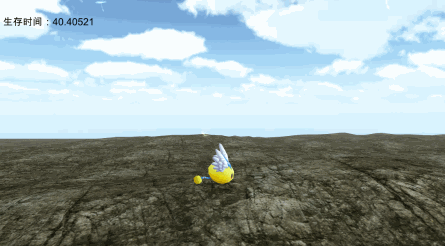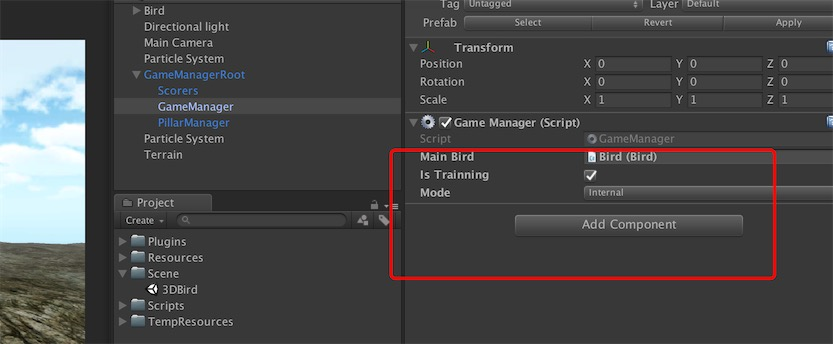// greedy police
float epsilon = 0.9f;

// learning rate
float alpha = 0.1f;

//discount factor
float gamma = 0.9f;




/// <summary>
/// Dictionary做二维表，key 是代表的状态，
/// Row 存储对应的 action 的 Q值
/// </summary>
Dictionary<int, Row> q_table;

public class Row
{
/// <summary>
/// 拍翅膀
/// </summary>
public float pad;

/// <summary>
/// 继续滑翔
/// </summary>
public float stay;
}


  /*
comment: tick time is 15f
*/
public void OnTick()
{
int state = GetCurrentState();
if (last_state != -1)
{
//cul last loop
UpdateState(last_state, state, last_r, last_action);
}

//do next loop
bool action = choose_action(state);
GameManager.S.RespondByDecision(action);
last_r = 1;
last_state = state;
last_action = action;
}



/// <summary>
/// 导出q_table
/// </summary>
public void exportQTable()
{
Debug.Log(save_path);
FileStream fs = new FileStream(save_path, FileMode.OpenOrCreate, FileAccess.Write);
StreamWriter sw = new StreamWriter(fs);
foreach (var item in q_table)
{
string line = item.Key + "," + item.Value.pad + "," + item.Value.stay;
sw.WriteLine(line);
}
sw.Close();
fs.Close();
}

/// <summary>
/// 游戏进入时 加载q_table
/// </summary>
private void loadQTable()
{
if (q_table == null) q_table = new Dictionary<int, Row>();
if (File.Exists(save_path))
{
FileStream fs = new FileStream(save_path, FileMode.Open, FileAccess.Read);
StreamReader sr = new StreamReader(fs);
while (true)
{
string line = sr.ReadLine();
if (string.IsNullOrEmpty(line)) break;
string[] ch = line.Split(':');
if (ch.Length >= 3)
{
int key = int.Parse(ch);
float pad = float.Parse(ch);
float stay = float.Parse(ch);
Row row = new Row() { stay = stay, pad = pad };
if (!q_table.ContainsKey(key)) q_table.Add(key, row);
else q_table[key] = row;
}
}
sr.Dispose();
fs.Dispose();
}
}



### 难度二：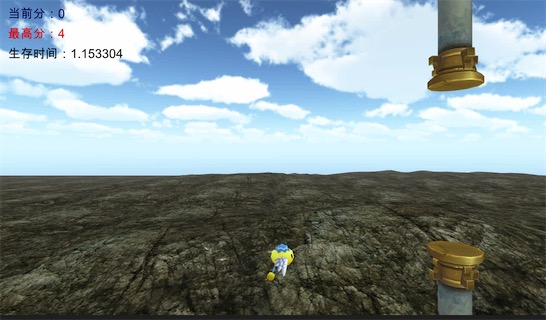public int GetPillarMiniState()
{
int ret = 0;
if (pillars.Count > 0)
{
float _dis = pillars.transform.position.x;
if (_dis < 0) ret = 0;
else if (_dis <= 2) ret = 1;
else if (_dis <= 4) ret = 2;
else if (_dis <= 6) ret = 3;
else ret = 4;
}
return ret * 10;
}



Pillar 和Bird 一共组合了9X5=45种状态， 我们在构建 q_table的时候，代码如下：

/// <summary>
/// Bird [0-9)一共九个状态
/// Pillar [0-5) 一共5个状态
/// 状态统计 9x5=45个状态
/// </summary>
public void Build_Q_Table()
{
q_table = new Dictionary<int, Row>();
for (int i = 0; i < 9; i++)
{
#if ENABLE_PILLAR
for (int j = 0; j < 5; j++)
{
Row row = new Row() { pad = 0f, stay = 0f };
Debug.Log("i:" + i + " j:" + j + " val:" + (i + 10 * j));
q_table.Add(i + 10 * j, row);
}
#else
Row row = new Row() { pad = 0f, stay = 0f };
q_table.Add(i, row);
#endif

}
}

public int GetCurrentState()
{
#if ENABLE_PILLAR
int p_st = PillarManager.S.GetPillarMiniState();
int b_st = GameManager.S.mainBird.GetState();
return p_st + b_st;
#else
return GameManager.S.mainBird.GetState();
#endif
}


Reinforcement做选择还是和之前一样，由 epsilon概率来由 q_table 来决定，1-epsilon概率随机决定。

### 难度三：